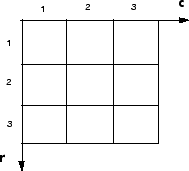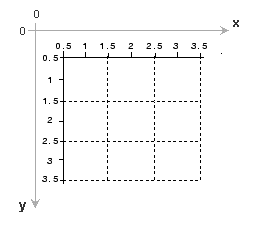## Image Coordinate Systems

You can access locations in images using several different image coordinate systems. You can specify locations using discrete pixel indices because images are stored as arrays. You can also specify locations using continuous spatial coordinates because images represent real-world scenes in continuous space.

### Pixel Indices

As described in Images in MATLAB, MATLAB® stores most images as arrays. Each (row, column) index of the array corresponds to a single pixel in the displayed image.

There is a one-to-one correspondence between pixel indices and subscripts for the first two matrix dimensions. Just like for array indexing in MATLAB, pixel indices are integer values and range from 1 to the length of the row or column. The indices are ordered from top to bottom, and from left to right.For example, the data for the pixel in the fifth row, second column is stored in the matrix element (5,2). You use normal MATLAB matrix subscripting to access values of individual pixels. For example, the MATLAB code

`I(2,15)`

returns the value of the pixel at row 2, column 15 of the single-channel image `I`. Similarly, the MATLAB code

`RGB(2,15,:)`

returns the color values of the pixel at row 2, column 15 of the multi-channel image `RGB`.

### Spatial Coordinates

In a spatial coordinate system, locations in an image are positions on a continuous plane. Locations are described in terms of Cartesian x and y coordinates (not row and column indices as in the pixel indexing system). From this Cartesian perspective, an (x,y) location such as (3.2,5.3) is meaningful and distinct from the coordinate (5,3).

The Image Processing Toolbox™ defines two types of spatial coordinate systems depending on the frame of reference. Intrinsic coordinates specify locations with respect to the image's frame of reference. World coordinates specify locations with respect to an external world observer.

#### Intrinsic Coordinates

By default, the toolbox defines spatial image coordinates using the intrinsic coordinate system. This spatial coordinate system corresponds to the image’s pixel indices. The intrinsic coordinates (x,y) of the center point of any pixel are identical to the column and row indices for that pixel. For example, the center point of the pixel in row 5, column 3 has spatial coordinates x = 3.0, y = 5.0. Be aware, however, that the order of order of intrinsic coordinate (3.0,5.0) is reversed relative to pixel indices (5,3).The intrinsic coordinates of the center of every pixel are integer valued. The center of the upper left pixel has intrinsic coordinates (1.0, 1.0). The center of the lower right pixel has intrinsic coordinates (numCols, numRows), where numCols and numRows are the number of rows and columns in the image. In general, the center of the pixel with pixel indices (m, n) falls at the point x = n, y = m in the intrinsic coordinate system.

Because the size of each pixel in the intrinsic coordinate system is one unit, the boundaries of the image have fractional coordinates. The upper left corner of the image is located at (0.5,0.5), not at (0,0). Similarly, the lower right corner of the image is located at (`numCols` + 0.5, `numRows` + 0.5).

Several functions primarily work with spatial coordinates rather than pixel indices, but as long as you are using the default spatial coordinate system (intrinsic coordinates), you can specify locations in terms of their columns (x) and rows (y).

#### World Coordinates

In some situations, you might want to use a world coordinate system (also called a nondefault spatial coordinate system). Some situations when you might want to use a world coordinate system include:

• When you perform a geometric operation, such as translation, on an image and want to preserve information about how the new position relates to the original position.

• When pixels are not square. For example, in magnetic resonance imaging (MRI), data you can collect data such that pixels have a higher sampling rate in one direction than an orthogonal direction.

• When you know how the extent of pixels aligns with positions in the real world. For example, in an aerial photograph, every pixel might cover a specific 5-by-5 meter patch on the ground.

• When you want to reverse the direction of the x-axis or y-axis. This is a common technique to use with geospatial data.

There are several ways to define a world coordinate system. You can use spatial referencing objects, which encode the location of the image in a world coordinate system, the image resolution, and how the image extent relates to intrinsic and world coordinates. You can also specify the maximum and minimum coordinate in each dimension. For more information, see Define World Coordinate System of Image.